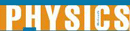# Resistance in Series and Parallel Circuits - Research Article from World of Physics

This encyclopedia article consists of approximately 3 pages of information about Resistance in Series and Parallel Circuits.
 This section contains 640 words (approx. 3 pages at 300 words per page) View a FREE sampleThe resistance of any electrical device is defined by the expression, R=V/I, where V (volts) is the potential difference across the device and I (amps) is the electrical current flowing through it.

When a current of one amp flows through a resistor after a potential of one volt is placed across it we say that the resistor has a unit resistance of one ohm. If the resistor is a metallic conductor then, as long as it's temperature is constant it's resistance remains constant. In other words, the ratio of potential difference (V) and current flow (I) remains constant. This is Ohm's Law. This means that for any given resistor doubling the potential potential across its ends will double the current flowing through it, tripling the potential will triple the current, etc.

The next interesting question is...

(read more)

 This section contains 640 words (approx. 3 pages at 300 words per page) View a FREE sampleCopyrightsResistance in Series and Parallel Circuits from Gale. ©2005-2006 Thomson Gale, a part of the Thomson Corporation. All rights reserved.